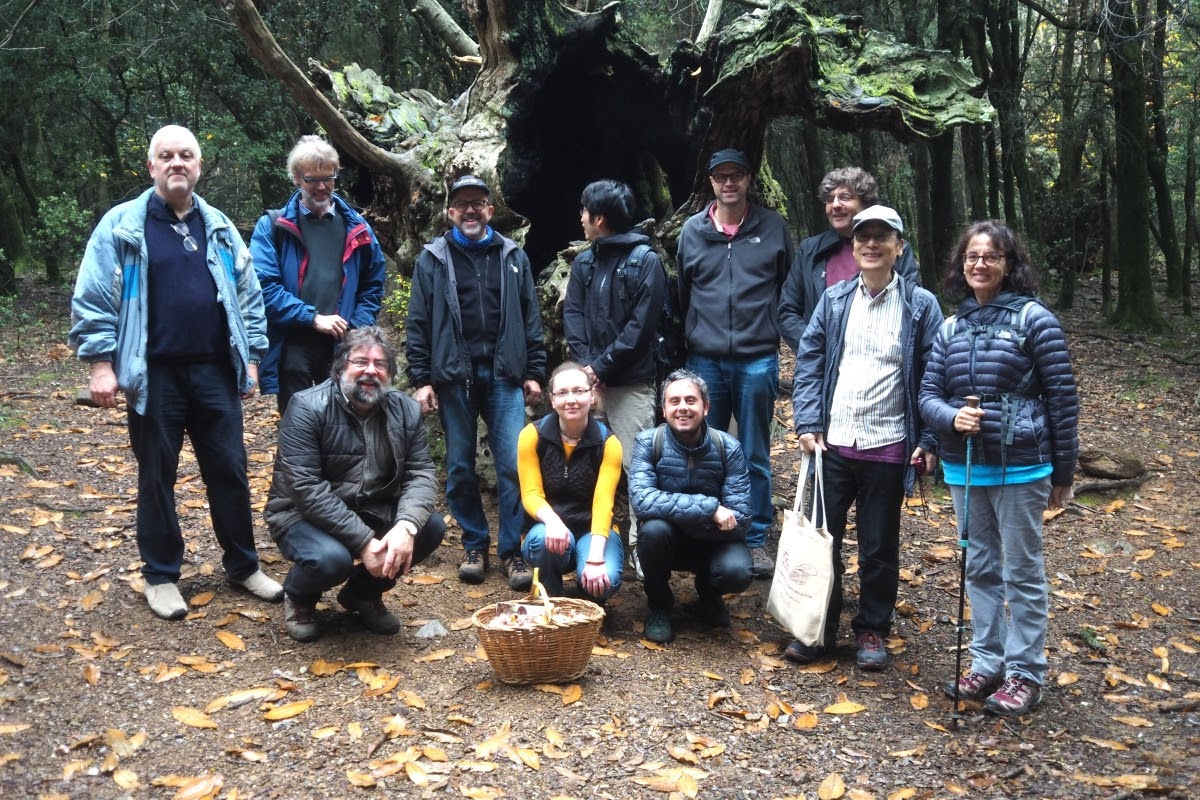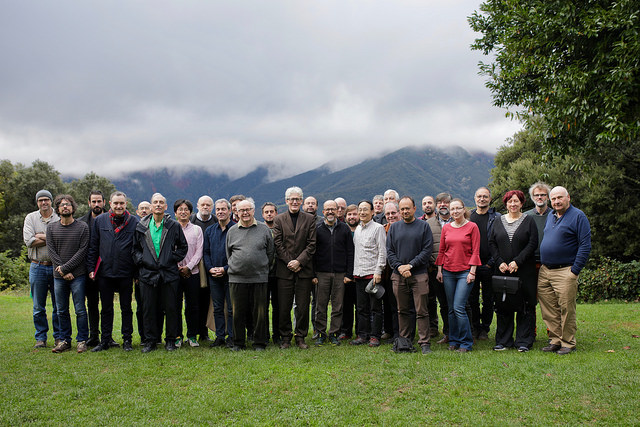# The Stable Core

This is a talk at the Reflections on Set Theoretic Reflection Conference, University of Barcelona, November 16-19, 2018.

Abstract: Sy Friedman introduced an inner model, which he called the stable core, in order to study under what circumstances the universe $V$ is a class forcing extension of ${\rm HOD}$. He showed there is a definable predicate $S$, amenable to ${\rm HOD}$, such that $V$ is a class forcing extension of the structure $\langle {\rm HOD},\in,S\rangle$. Namely, there is an ${\rm ORD}$-cc class partial order $\mathbb P$ definable in $\langle {\rm HOD},\in,S\rangle$ and a generic filter $G\subseteq \mathbb P$ such that ${\rm HOD}[G]=V$, however the filter $G$ itself is not definable over $V$. The predicate $S$, consisting of triples $(\alpha,\beta,n)$ for $\alpha$ and $\beta$ strong limit cardinals and $n\geq 1$ such that $H_\alpha\prec_{\Sigma_n}H_\beta$ and $\Sigma_n$-collection holds in $H_\beta$, codes elementarity relations between nice enough initial segments $H_\alpha$ of $V$. Friedman's argument showed that the information necessary to define $\mathbb P$ is already contained in $S$ so that $V$ is already a class forcing extension of the stable core $\langle L[S],\in,S\rangle$.

In a joint work with Friedman and Sandra Müller, we investigated the properties of the stable core. We were interested to see whether the stable core is in any sense a canonical inner model, whether it has regularity properties, whether it is consistent with large cardinals, and whether we can code information into it using forcing. We showed that the stable core of $L[\mu]$, the canonical model for a single measurable cardinal, is $L[\mu]$ and therefore measurable cardinals are consistent with the stable core. By coding generic sets into the stable core over $L$ or $L[\mu]$, we showed that there is a generic extension of $L$ in which the ${\rm GCH}$ fails at every regular cardinal in the stable core and there is a generic extension of $L[\mu]$ in which there is a measurable cardinal which is not even weakly compact in the stable core. Our work leaves numerous open questions about the structure of the stable core in the presence of large cardinals beyond a measurable cardinal.